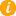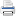Math K-Plus

# Practice 1st Grade Subtraction Calculator

TestQuizQuiz Results1st Grade Subtraction calculator has an extra feature. Not only is a complete numerical answer shown for each math problem, a visual answer is also shown; this helps students transition from thinking visually to thinking numerically.

• Option 2. Solve a calculator generated math problem. First press the NEW PROBLEM button. Next enter your answer, in to the calculator. Lastly, press the ADD button and then compare your answer with the calculator's.

Field Name Subtract Values
Minuend: You can enter only 1 digit
Subtrahend: You can enter only 1 digitAfter learning First Grade Subtraction Calculator, you can move to the next step in subtraction math: Second Grade Subtraction Calculator.

+7
-  6
————

7
-  6
————

7
-  6
————
1

7
-  6
————
+1